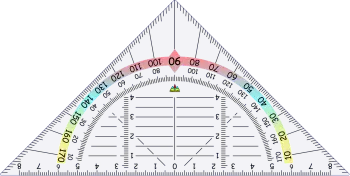# What Are the Angles? Classification, Types and NamesAngles are fundamental geometric figures in various branches of mathematics, particularly geometry.

Angles indicate the difference in inclination between two lines or two planes and is measured in degrees or radians. In geometry, we refer to this concept to refer to the set that forms the vertex and the two edges that form it.

In geometry there are many figures that are defined according to the shape and dimension of their angles. For example, regular polygons have all angles equal. Among other regular polygons we can highlight the square (with all its edges at ninety degrees), the pentagon, or the equilateral triangle.

## What Are the Angles?

Angles are a measure that indicates the inclination of a straight line with respect to another. In three-dimensional space, they are also used to determine the difference in inclination between two planes.

When we talk about geometric figures, an angle is the part of the figure made up of two sides that intersect at a point called the vertex. For example, a square is made up of four angles that measure 90 degrees on the inside and 270 degrees on the outside.

This measurement can be expressed in degrees or radians. A circle is divided into 360º or 2π radians forming a complete angle.

## Angle Classification

These geometric elements can be classified in different ways:

• The angles according to their position between them can be classified as: adjacent, consecutive, opposite angles by the vertex, interior and exterior of a geometric figure.

• According to the arithmetic relations between them. In this sense, they can be congruent, complementary, supplementary or conjugated.

• Determined by two parallels and a transversal: these can be parallel lines cut by a secant, corresponding and alternate.

• The angles according to their measure are differentiated by the position of their ends and can be: right, acute, obtuse and flat.

## What Are the Types of Angles?

• The right angle is the one that has a measure of 90°, and is formed when the two lines intersect perpendicularly.

• An acute angle is one that has a measure less than 90°.

• An obtuse angle is one that has a measure greater than 90° but less than 180°.

• The straight or flat angle is the one that has a measure equal to 180 °.

Depending on the relationship they have with other angles they receive the following names:

• Complementary angles: the sum of their degrees is equal to 90º.

• Consecutive: are angles that share a vertex and a side.

• Adjacent angles: they also share a vertex and a side but also their two add up to 180º.

• Convex angle: is between 0 and 180 degrees.

• Concave angle: It is more than 180 degrees and therefore not convex.

• Interior: The interior angle is the angle between two consecutive edges of a closed geometric figure measured from the interior of the figure.

• Exterior: is formed by two consecutive sides of a figure measured from the outside of the figure.

## Formula to Convert Radians to Degrees

Angles can be measured in degrees or radians. To convert from one unit to another, we use the formula:

• degrees = radians * 180 / π.

• radians = degrees * π / 180.

A complete circle consists of 360 degrees and 2π radians. This means that 1 radian is equal to 360 / 2π = 57.29578 degrees. Therefore, we can say that:

• 1 degree = π / 180 ≈ 0.0174 radians.

• 1 radian ≈ 57.29578 degrees.

Author:

Published: February 8, 2023
Last review: February 8, 2023